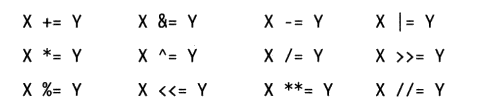### 文章目录

• 一、什么是赋值
• 二、赋值类型
• 1. 序列赋值
• 2. 多目标赋值
• 3. 增强赋值语句

## 一、什么是赋值

python的赋值是通过“`=`” 将右边的`对象`赋值给左边的`变量`，如：

``````a = 'test'		#将字符串赋值给变量a
a = 123			#将数值赋值给变脸a
a = [1,2,3]		#将列表赋值给变量a
``````
• 1
• 2
• 3

1. 赋值的过程是建立变量到对象的引用的过程
2. 变量在首次赋值时被创建
3. 变量在引用前必须先赋值

## 二、赋值类型

### 1. 序列赋值

python将`=`右侧序列的值依次和左侧的序列中的变量相互对应匹配，给每一个变量赋值，如：

``````a,b,c = [1,2,3]
a,b,c=(1,2,3)
(a,b,c)=1,2,3
(a,b,c)=[1,2,3]
a,b,c=1,2,3
*a,b = 1,2,3	#首先满足非*的变量，剩下的通过列表复制给待*的变量，如果没有剩下的值，那么列表为空。其典型应用详见小技巧4.
``````
• 1
• 2
• 3
• 4
• 5
• 6

``````a,b=1,2,3
ValueError: too many values to unpack (expected 2)
``````
• 1
• 2

``````a,b,c,d,e = 'hello'

dct = {'a':1, 'b':2, 'c':3}
a,b,c = dct		#只是将字典中的key 复制给a,b,c
``````
• 1
• 2
• 3
• 4

``````a = 1
b = 1
a,b =b,a		#完成a，b值的交换
``````
• 1
• 2
• 3

``````a,b,c,d,e,f = range(6)
``````
• 1

``````a,b,c=1,2,3,4		#会报错
a,b,*c=1,2,3,4				#c为[3,4]，a，b正常赋值
a,*b,c=1,2,3,4				#b为[2,3]，a，c正常赋值
*a,b,c=1,2,3,4				#a为[1,2]，b，c正常赋值
a,b,c,d,*e=1,2,3,4			#e将得到一个空列表
``````
• 1
• 2
• 3
• 4
• 5

``````P = [1, 10, 7, 4, 5, 9]			#求列表P中个元素之和

def sum_p(items):

sum_p(P)	#output: 36
``````
• 1
• 2
• 3
• 4
• 5
• 6
• 7

### 2. 多目标赋值

``````a=b=c=d=e=0
``````
• 1

### 3. 增强赋值语句

X += Y 本质是 X = X + Y,先求X+Y的和，再将其赋值给X